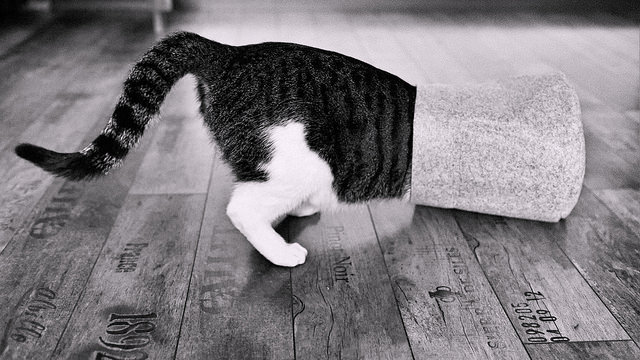# Quick Steganography with Matlab

|## Overview

Although there are many ways to conceal information within media file types, in this blog I want to talk about implementing two such techniques in Matlab:

1. The well-known LSB technique.
2. A lesser known but very interesting technique using DCT quantization quotients from JPEG compression algorithms.

This research is part of a project to implement eraseable and semi-fragile watermarks using Matlab, and the full source code for all the modules is available here.

## LSB (Least Significant Bit) Steganography

The concept of using the last significant bit of something is very generic, and applies not only to RGB bitmaps, but to many kinds of images, audio, video, etc. Implementation of this is very straigh-forward. First we have the module that hides the data inside BMP images - bmpHide.m:

``````%%%%%%%%%%%%%%%%%%%%%%%%%%%%%%%%%%%%%%%%%%%%%%%%%%%%%%%%%%%%%%%%%%%%%%%%%%%
% Simple function to hide data in LSB coefficients in BMPs.
% (Working for common 24bit depth bmp images)
%
% Arguments:
%   original [IN] - input image filename
%   msg [IN] - message string to be hidden
%   stego [OUT] - output image filename with embedded message
%
% Return:
%   0 if entire message was hidden succesfully
%
%%%%%%%%%%%%%%%%%%%%%%%%%%%%%%%%%%%%%%%%%%%%%%%%%%%%%%%%%%%%%%%%%%%%%%%%%%%

function ret = bmpHide(original, msg, stego)
% Log file
log = fopen('bmpHide.log', 'w');

% Convert message string to binary representation
bin_msg = msg - 0;
bin_msg = reshape(de2bi(bin_msg, 8), 1, length(msg) * 8);

% Add message size on 32 bits
MAX_SIZE_BITS = 32;
bin_msg = horzcat(de2bi(length(bin_msg), MAX_SIZE_BITS), bin_msg);

len_m = length(bin_msg);

% Use the R matrix
r = im(:, :, 1);
[w,h] = size(r);

cnt = 1;
for i=1:w
for j=1:h
color = r(i,j);

if (cnt <= len_m)
% Embed a bit here
color = bitset(color, 1, bin_msg(cnt));

fprintf(log, 'color(%d, %d) before: %d, bit %d: %d after: %d\r\n', ...
i, j, r(i,j), cnt, bin_msg(cnt), color);

cnt = cnt + 1;
r(i,j) = color;
end
end
end

if (cnt == len_m + 1)
fprintf(log, 'All %d bits embedded\r\n', len_m);
ret = 0;
else
fprintf(log, 'Embedded %d of %d bits\r\n', cnt-1, len_m);
ret = 1;
end

% Write modified BMP to output file
im(:, :, 1) = r;
imwrite(im, stego);

fclose(log);
end
``````

To extract the data we have a similar module - bmpExtract.m:

``````%%%%%%%%%%%%%%%%%%%%%%%%%%%%%%%%%%%%%%%%%%%%%%%%%%%%%%%%%%%%%%%%%%%%%%%%%%%
% Simple function to extract hidden data from LSB coefficients of BMPs.
%
% Arguments:
%   stego [IN] - input image containing message
%
% Return:
%   extracted message
%
%%%%%%%%%%%%%%%%%%%%%%%%%%%%%%%%%%%%%%%%%%%%%%%%%%%%%%%%%%%%%%%%%%%%%%%%%%%

function msg = bmpExtract(stego)
% Log file
log = fopen('bmpExtract.log', 'w');

MAX_SIZE_BITS = 32;

cnt = 1;
len_m = zeros(1, 32); % Bits representing size of the embedded message
size_m = 0;
emb_m = []; % Embedded message

% Use the R matrix
r = im(:, :, 1);
[w,h] = size(r);

for i=1:w
for j=1:h
color = r(i,j);

if (size_m == 0)
% Message size not yet caculated
if (cnt <=  MAX_SIZE_BITS)
len_m(cnt) = bitget(color, 1);
cnt = cnt + 1;

if (cnt == MAX_SIZE_BITS + 1)
size_m = bi2de(len_m);
fprintf(log, 'Got size %d\r\n', size_m);
emb_m = zeros(1, size_m);
cnt = 1;
end
end
else
if (cnt <= size_m)
emb_m(cnt) = bitget(color, 1);
fprintf(log, 'Color(%d, %d) got bit %d: %d\r\n', ...
i, j, cnt, emb_m(cnt));
cnt = cnt + 1;
else
break;
end
end

end
end

% Convert message to string
emb_m = reshape(emb_m, size_m/8, 8);
msg = zeros(1, size_m/8); % characters in the retrieved message

for k=1:size_m/8
msg(k) = bi2de(emb_m(k, :));
end

msg = char(msg);

fclose(log);

end
``````

## DCT Steganography

• This idea of hiding information in DCT coefficients is implemented by the JSTEG tool, which is the software from Independent JPEG Group JPEG, modifed for 1-bit steganography, developed by Derek Upham. Its source is readily available.

The JPEG encoding procedure divides an image into 8x8 blocks of pixels in the YCbCr colorspace. Then they are run through a discrete cosine transform (DCT) and the resulting frequency coefficients are scaled to remove the ones which a human viewer would not detect under normal conditions. If steganographic data is being loaded into the JPEG image, the loading occurs after this step. The lowest-order bits of all non-zero frequency coefficients are replaced with successive bits from the steganographic source file, and these modified coefficients are sent to the Huffmann coder.